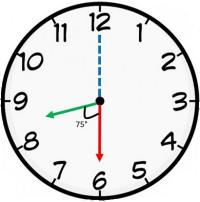# Clocks - Logical Reasoning (MCQ) Questions for Q. 30022

Q.  The angle between the minute hand and the hour hand of a clock when the time is 8.30, is
- Published on 15 May 17

a. 65
b. 75
c. 85
d. 90

At 8 o’ clock, the hour hand is at 240 degrees from the vertical.

In 30 minutes,
Hour hand = 240 + 30 x (0.5) = 240 + 15 = 255 (The hour hand moves at 0.5 dpm)

Minute hand = 30 x (6) = 180 (The minute hand moves at 6 dpm)

Difference or angle between the hands = 255 - 180 = 75 degrees.## ➨ Post your comment / Share knowledgeEnter the code shown above:

(Note: If you cannot read the numbers in the above image, reload the page to generate a new one.)